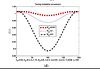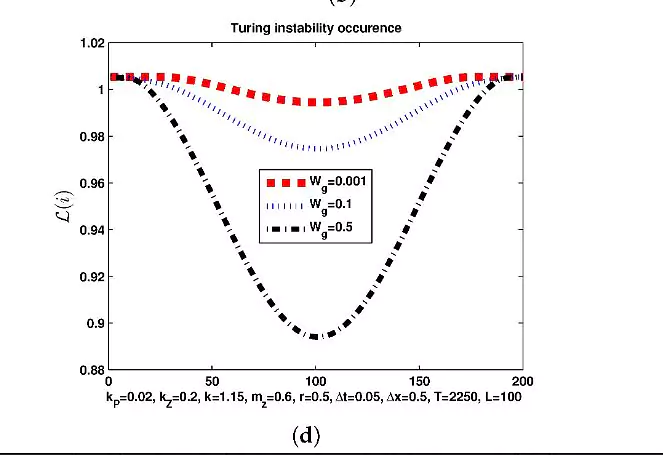# Fasma Diele# One-step methods for ODEs in MATLAB

One-step methods for the solution of ordinary differential equations with Matlab

Docente: dott.ssa Fasma Diele

• Introduction: existence, uniqueness and well-posedness. Examples and test problem solutions with the Matlab routine “dsolve”.

• ODE discretization: local truncation error, error propagation. Consistency, zero-stability and convergence of a one-step method.

• Explicit and Implicit Euler method, Theta method, Trapezoidal Rule. Convergence analysis. Implementation of methods and solution of test problems writing  Matlab routines.

• Quadrature methods and explicit Runge-Kutta methods. Order conditions and Butcher’s barrier. Heun’s method and classical fourth-order method. Implementation of methods and solution of test problems writing  Matlab routines.

• Implicit and diagonally-implicit Runge-Kutta methods. Collocation methods: Gauss, Radau IIA, Lobatto IIIA methods. Implicit Midpoint rule. Solution of nonlinear systems  with Matlab routine “fsolve”. Implementation of Gauss methods and solution of test problems writing  Matlab routines.

• Variable stepsize methods: error estimation and stepsize selection. Runge-Kutta embedded methods: DOPRI45 e BS(2,3). Implementation of methods and solution of test problems with Matlab routines “ode45” and “ode23”.

• Hints on linear stability and regions of absolute stability  of Runge-Kutta methods.

• Conservative methods: symplectic methods for Hamiltonian systems.

• Symplectic and Partitioned Runge-Kutta methods: Symplectic Euler and Stӧrmer/Verlet method. Implementation of methods and solution of test problems writing  Matlab routines.

• Composition methods, splitting methods and splitting and composition methods for autonomous separable problems. Equivalence between symplectic and composition methods and symplectic partitioned Runge-Kutta methods.

Bibliografia

1. E. Hairer, S.P. Norsett, G. Wanner, Solving ordinary differential equations I, Nonstiff Problems, Springer-Verlag, Berlin, 1987
2. E. Hairer, C. Lubich, G.Wanner, Geometric Numerical Integration, Springer, Berlin, 2002
3. A. Iserles, A First Course in the Numerical Analysis of Differential Equations, Cambridge University Press, Cambridge, 1996
4. J.D. Lambert, Numerical Methods for Ordinary Differential Systems, Wiley, Chichester, 1991
5. L.F. Shampine, I. Gladwell, S. Thompson, Solving ODEs with Matlab, Cambridge, 2003.
Share